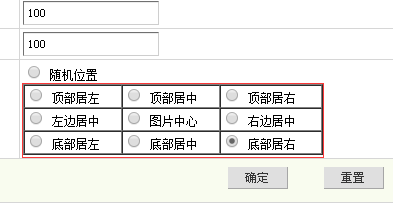x = +5;
\$y = +5;
break;
case 2:
\$x = (\$imagewidth - \$logowidth) / 2;
\$y = +5;
break;
case 3:
\$x = \$imagewidth - \$logowidth - 5;
\$y = +5;
break;
case 4:
\$x = +5;
\$y = (\$imageheight - \$logoheight) / 2;
break;
case 5:
\$x = (\$imagewidth - \$logowidth) / 2;
\$y = (\$imageheight - \$logoheight) / 2;
break;
case 6:
\$x = \$imagewidth - \$logowidth - 5;
\$y = (\$imageheight - \$logoheight) / 2;
break;
case 7:
\$x = +5;
\$y = \$imageheight - \$logoheight - 5;
break;
case 8:
\$x = (\$imagewidth - \$logowidth) / 2;
\$y = \$imageheight - \$logoheight - 5;
break;
case 9:
\$x = \$imagewidth - \$logowidth - 5;
\$y = \$imageheight - \$logoheight -5;
break